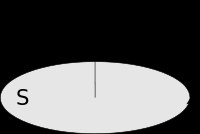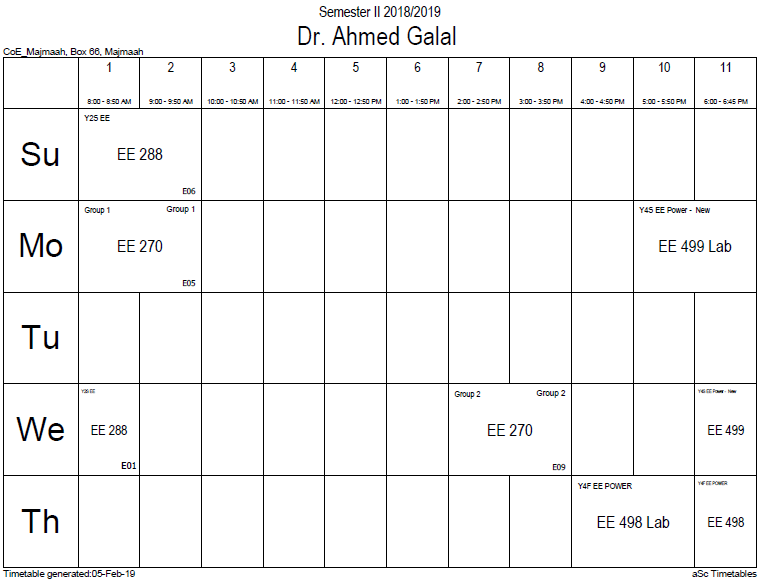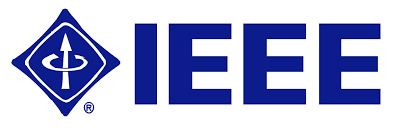## Current loop defin

Suppose a planar closed loop carries an electric current I and has vector area S (x, y, and z coordinates of this vector are the areas of projections of the loop onto the yz, zx, and xy planes). Its magnetic moment m, vector, is defined as:$mathbf{m}=I mathbf{S}.$

By convention, the direction of the vector area is given by the right hand grip rule (curling the fingers of one's right hand in the direction of the current around the loop, when the palm of the hand is "touching" the loop's outer edge, and the straight thumb indicates the direction of the vector area and thus of the magnetic moment).

If the loop is not planar, the moment is given as$mathbf{m}=frac{I}{2}intmathbf{r} imes{ m d}mathbf{r}.$

where × is the vector cross product. In the most general case of an arbitrary current distribution in space, the magnetic moment of such a distribution can be found from the following equation:$mathbf{m}=frac{1}{2}intmathbf{r} imesmathbf{J},{ m d}V,$

where r is the position vector pointing from the origin to the location of the volume element, and J is the current density vector at that location.

The above equation can be used for calculating a magnetic moment of any assembly of moving charges, such as a spinning charged solid, by substituting$mathbf{J}= ho mathbf{v},$

where ρ is the electric charge density at a given point and v is the instantaneous linear velocity of that point.

For example, the magnetic moment produced by an electric charge moving along a circular path is$mathbf{m}=frac{1}{2}, q, mathbf{r} imesmathbf{v}$,

where r is the position of the charge q relative to the center of the circle and v is the instantaneous velocity of the charge.

Practitioners using the current loop model generally represent the magnetic field by the solenoidal field B, analogous to the electrostatic field D.### Office HoursNo office hours### My Timetable### Contactsemail: [email protected]

Phone: 2570

### Welcome

Welcome To Faculty of Engineering### IEEEhttp://www.ieee.org/

/

### Bookmarkshttp://www.utk.edu/research/

http://science.doe.gov/grants/index.asp

http://www1.eere.energy.gov/vehiclesandfuels/

http://www.eere.energy.gov/

### Upcoming Conferences### Engineering quotes### Travel Web Siteshttp://www.hotels.com/

http://www.orbitz.com/

http://www.hotwire.com/us/index.jsp

http://www.kayak.com/

### Blackboardستقام اختبارات الميدتيرم يوم الثلاثاء 26-6-1440

حسب الجدول المعلن بلوحات الاعلان

### Summer trainingThe registration for summer training will start from 5th week of second semesterClass registration week 1

### برنامج التجسير### إحصائية الموقع

عدد الصفحات: 2879

البحوث والمحاضرات: 1280

الزيارات: 99769